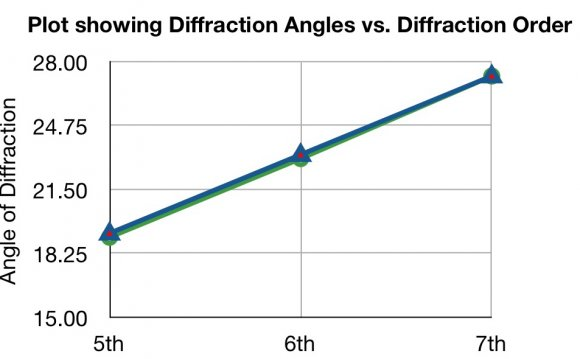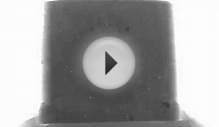# Angles of diffraction

October 6, 2015Let L represent the distance between the slit and the screen.
Let T represent the angle between the wave ray to a point on the screen
and the normal line between the slit and the screen.

The top part of the figure to the left is an imitation of a single slit diffraction pattern which may be observed on the screen (there would really be more blending between the bright and dark bands, see a at the top of this page).

Below the pattern is an intensity bar graph showing the intensity of the light in the diffraction pattern as a function of sin T.

Most of the light is concentrated in the broad CENTRAL DIFFRACTION MAXIMUM.
There are minor seconday bands on either side of the central maximum.

The first DIFFRACTION MINIMUM occurs at the angles given by sin T = l / a

I will mention now that the intensity of light is proportional to the square of its amplitude. This will come into play later on.

With the equation:

sin T = l / a (*)

note that the width of the central diffraction maximum is inversely proportional to the width of the slit. If we increase the width size, a, the angle T at which the intensity first becomes zero decreases, resulting in a narrower central band. And if we make the slit width smaller, the angle T increases, giving a wider central band.

But why are there these bands of light?
And how can we derive the equation (*) for the location of the central diffraction minimum?

The equation (*) is the result of analysis of the PATH DIFFERENCE between light rays coming from the top and the bottom of the slit, and how this path difference relates to our discussion on INTERFERENCE.

Recall that we are considering points within the aperature as point sources from which new waves spread out. In the diagrams below the waves have been drawn from a side view, rather than a top view of wavefronts. This is to help us compare the phase of the waves.

At T = 0, when the wave rays follow the normal line directly to the screen, a sin T = 0. This means that the path difference and the phase difference of all the waves is zero. Hence the waves are all in phase, and constructive interference has the resultant wave's amplitude equal to the sum of all the individual wave's amplitudes.

This explains the very bright central band around sin T = 0. With all the waves in phase, we have the largest resultant wave amplitude possible. And since the intensity of light is proportional to the square of its amplitude, the pattern on the screen has a very intense central band at this angle, that is when T = 0.

As T varies slightly from zero, a sin T also varies slightly from zero, as does the phase difference of the waves. This results in the interference of all the waves being not totally constructive, and so the intesity of the central band decreases while moving slightly away from sin T = 0, as we saw in the intesity bar graph above.

Let y be the distance from the center of the central diffraction maximum to the first diffraction minimum.

The angle T is related to this distance y and the distance to the screen, L, by the equation:

tan T = y / L

Since the angle T is very small, cos T » 1. Thus, tan T » sin T. Then, combining the above equation with equation (*), we have sin T = l / a » y / L, or

y = L l / a

And so, given the distance to the screen, the width of the slit, and the wavelength of the light, we can use the equation y = L l / a to calculate where the first diffraction minimum will occur in the single slit diffraction pattern. And we have learned that this is the point where the waves from point sources in the slit all cancel in pairs that are out of phase.

RESOURCES:
I must mention here my first year physics textbook:
Tipler, Paul A. Physics for Scientists and Engineers. 4th Ed. New York: W.H. Freeman and Company, 1999.

Source: www.math.ubc.ca
##### RELATED VIDEODiffraction of graphic quartz crystals.Diffraction - Swarm of Angels (Sceptis Ep)Physics - Diffraction of Light (3 of 4) The Diffraction ...